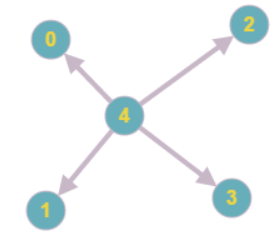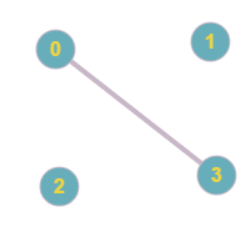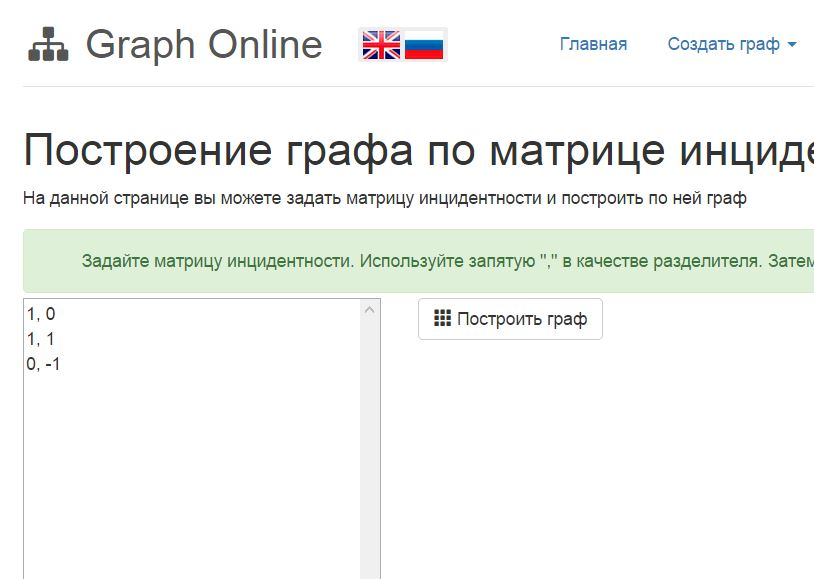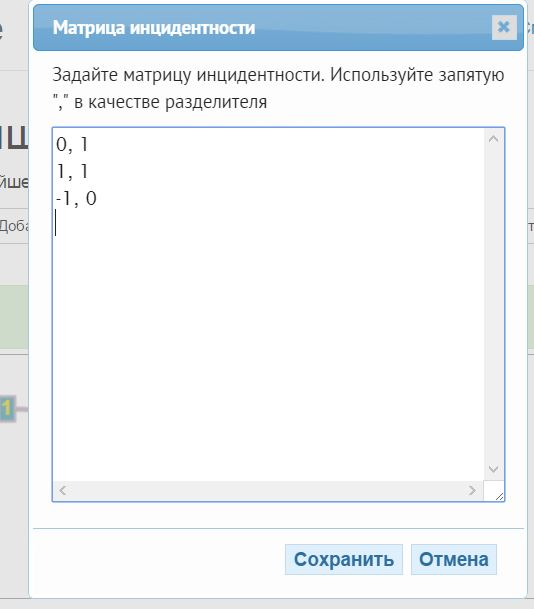Help

# Incidence Matrix

Incidence matrix represents a graph in the form of matrix, where every column defines a separate edge. Meanwhile, the matrix's rows define the vertices. A positive number defines a vertex, where an edge starts; whereas a negative number defines a vertex, where an edge finishes. If both numbers are positive, then the edge is non-oriented.

## Examples of the incidence matrices

Graph consisting of3 conjoined vertices Graph with oriented edges Graph with 4 vertices and one edge
```
1, 0, -3
-1, 1,  0
0, 1,  3
```
```-1,  0,  0,  0
0,  0,  0, -1
0, -1,  0,  0
0,  0, -1,  0
1,  1,  1,  1
```
```1
0
0
1
```Ссылка на граф Ссылка на граф Ссылка на граф

## Incidence matrix in Graph Online

Graph Online provides you with possibility to create Создать граф по матрице инцидентности.Moreover, you can edit the existing incidence matrix. You have to choose Graph in the menu bar and then Incidence matrix.In order to use incidence matrix, you have to enter it in the right form.

### The form for entering incidence matrix

Entering an incidence matrix you have to take into account the following principles:

1. The matrix should contain the number of rows equal to the number of vertices and the rows equal to the number of the edges.
2. In order to define non-oriented edge, you have to put their weight into the rows corresponding to starting and finishing vertices.
3. In order to define an oriented edge, you have to put its weight into the row corresponding to the starting vertex; and you have to put its weight with negative meaning into the rows corresponding to the finishing vertex.
Right matrix
```
0,1,0
0,0,1
0,0,1
0,0,1
```
In the first column starting and finishing vertices are not enterd. In the row 3 the edge joining 3 vertices was entered, such a format is not supported.
```
1,0,0,0
0,1,0,1
0,1,1,0
0,0,1,1
```
```
1, 0, 0
1, 1
0,-1
```
Last "0" in the first row is excess
```
1, 0
1, 1
0,-1
```